Next: TIME-STATISTICAL RESOLUTION Up: Resolution Previous: Resolution

# TIME-FREQUENCY RESOLUTION

The famous uncertainty principle'' of quantum mechanics resulted from observations that subatomic particles behave like waves with wave frequency proportional to particle momentum. The classical laws of mechanics enable prediction of the future of a mechanical system by extrapolation from presently known position and momentum. But because of the wave nature of matter with momentum proportional to frequency, such prediction requires simultaneous knowledge of both the location and the frequency of a wave. A sinusoidal wave has a perfectly clearly determined frequency, but it is spread over the infinitely long time axis. At the other extreme is a delta function, which is nicely compressed to a point on the time axis but contains a mixture of all frequencies. A mathematical analysis of the uncertainty principle is thus an analysis relating functions to their Fourier transforms.

Such an analysis begins by definitions of time duration and spectral bandwidth. The time duration of a damped exponential function is infinite if by duration you mean the span of nonzero function values. However, for nearly all practical purposes the time span is chosen as the time required for the amplitude to decay to e-1 of its original value. For many functions the span is defined by the span between points on the time or frequency axis where the curve (or its envelope) drop to half of the maximum value. The main idea is that the time span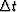or the frequency span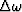should be able to include most of the total energy but need not contain all of it. The precise definition ofandis somewhat arbitrary and may be chosen to simplify analysis. The general statement is that for any function the time durationand the spectral bandwidthare related by(1)
Although it is easy to verify (1) in many special cases, it is not very easy to deduce (1) as a general principle. This has, however, been done by D. Gabor. He chose to defineandby second moments.

A similar and perhaps more basic concept than the product of time and frequency spreads is the relationship between spectral bandwidth and rise time of a system response function. The rise timeof a system response is also defined somewhat arbitrarily, often as the time span between the time of excitation and the time at which the system response is half its ultimate value. In principle, a broad frequency response can result from a rapid decay time as well as from a rapid rise time. Tightness in the inequality (1) may be associated with situations in which a certain rise time is quickly followed by an equal decay time. Slackness in the inequality (1) may be associated with increasing inequality between rise time and decay time. Slackness could also result from other combinations of rises and falls such as random combinations. Many systems respond very rapidly compared to the rate at which they subsequently decay. Focusing our attention on such systems, we can now seek to derive the inequality (1) applied to rise time and bandwidth. The first step is to choose a definition for rise time. The choice is determined not only for clarity and usefulness but also by the need to ensure tractability of the subsequent analysis. I have found a reasonable definition of rise time to be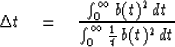(2)
where b(t) is the response function under consideration. The numerator is just a normalizing factor. The denominator says we have definedby the first negative moment. For example, if b(t) is a step function, then the denominator integral diverges, giving the desired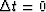rise time. If b(t)2 grows linearly from zero to t0 and then vanishes, the rise timeis t0/2, again a reasonable definition.

Although the Z transform method is a great aid in studying situations where divergence (as 1/t) plays a key role, it does have the disadvantage that it destroys the formal identity between the time domain and the frequency domain. Presumably this disadvantage is not fundamental since we can always go to a limiting process in which the discretized time domain tends to a continuum. In order to utilize the analytic simplicity of the Z transform we now consider the dual to the rise-time problem. Instead of a time function whose square vanishes identically at negative time we now consider a spectrumwhich vanishes at negative frequencies. We measure how fast this spectrum can rise after.We will find this to be related to the time durationof the complex time function bt. More precisely, we will now define the lowest significant frequency component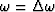in the spectrum analogously to (2) to be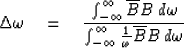(3)
Without loss of generality we can assume that the spectrum has been normalized so that the numerator integral is unity. In other words, the zero lag of the auto-correlation of bt is +1. Then(4)
Now we recall the bilinear transform which gives us various Z transform expressions for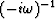.The one we ordinarily use is the integral.The pole right on the unit circle at Z = 1 causes some nonuniqueness. Becauseis an imaginary odd frequency function we will take the desired expansion to be the odd function of time given by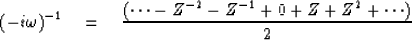(5)
Converting (4) to an integral on the unit circle in Z transform notation we have(6)
But since this integral selects the coefficient of Z0 of its argument we have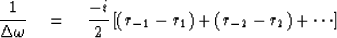(7)
where rt is the autocorrelation function of bt. This may be further expressed as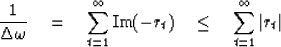(8)
The sum in (8) is like an integral representing area under the |rt| function. Imagine the |rt| function replaced by a rectangle function of equal area. This would define afor the |rt| function. Any autocorrelation function satisfies |rt| < r0 and we have normalized r0 = 1. Thus, we extend the inequality (8) by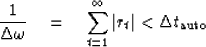(9)
Finally, we must relate the duration of a time functionto the duration of its autocorrelation.Generally speaking, it is easy to find a long time function which has short autocorrelation. Just take an arbitrary short time function and convolve it by a long and tortuous all-pass filter. The new function is long, but its autocorrelation is short. If a time function has n nonzero points, then its autocorrelation has only 2n -1 nonzero points. It is obviously impossible to get a long autocorrelation function out of a short time function. It is not even fair to say that the autocorrelation must lie under some tapering function. To construct a time function with as long an autocorrelation as possible, the best thing to do is to concentrate the energy in two lumps, one at each end of the time function. Even from this extreme example, we see that it is not unreasonable to assert that(10)
inserting into (9) we have the uncertainty relation(11)
The more usual form of the uncertainty principle uses the frequency variableand a different definition of, namely time duration rather than rise time. It is(12)
The choice of a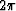scaling factor to convert rise time to duration is indicative of the approximate nature of the inequalities.

## EXERCISES:

1. Consider B(Z) = [1 - (Z/Z0)n]/(1 -Z/Z0) in the limit Z0 goes to the unit circle. Sketch the time function and its squared amplitude. Sketch the frequency function and its squared amplitude. Choose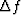and.
2. A time series made up of two frequencies may be written asGiven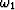,, b0, b1, b2, b3 show how to calculate the amplitude and phase angles of the two sinusoidal components.
3. Consider the frequency function graphed below.

 E4-1-3 Figure 1 Exercise 4.1.3.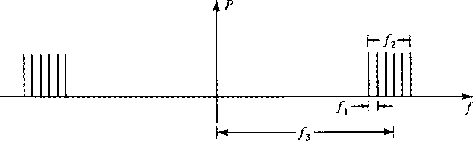Describe the time function in rough terms indicating the times corresponding to 1/f1, 1/f2, 1/f3. Try to avoid algebraic calculation. Sketch an approximate result.

PROBLEM FOR RESEARCH

Can you find a method of definingandof one-sided wavelets in such a way that for minimum-phase wavelets only the uncertainty principle takes on the equality sign?Next: TIME-STATISTICAL RESOLUTION Up: Resolution Previous: Resolution
Stanford Exploration Project
10/30/1997